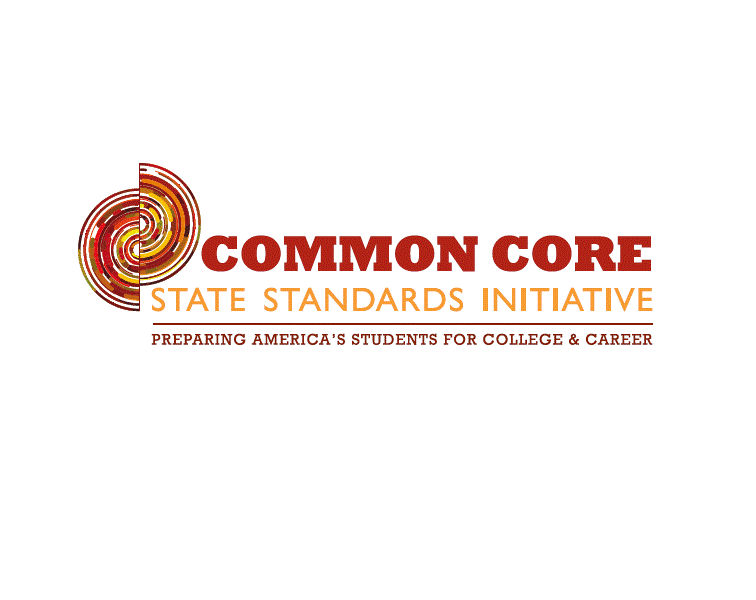AtReks math apps rely on on common core math standards in the U.S.A.  They are reliable follow-up resources for common core standardized lessons. AtReks math apps include math facts covering kindergarten through 3rd grade school curriculum. They are all based on the finding of the National Research Council:

Mathematics experiences in early childhood settings should concentrate on(1) number (which includes whole number, operations, and relations) and (2)

geometry, spatial relations, and measurement, with more mathematics learning

time devoted to number than to other topics. Mathematical process goals

should be integrated in these content areas.

—           Mathematics Learning  in Early Childhood, National Research Council, 2009

Here are some math skills that are expected from students to achieve towards the end of each grade level:

–        Kindergarten students should know whole numbers, count sequence by ones and by tens, compare numbers; they should understand addition and subtraction.

–        1st grade students should know whole numbers to 100, count sequence by twos and fives; they should develop strategies for adding and subtracting whole numbers based on their prior work with small numbers; they should use addition and subtraction within 20 to solve word problems.

–        2nd grade students should use their understanding of addition to develop fluency with addition and subtraction within 100; they should understand place value, as well as multiplication process/division.

–        3rd grade students should represent and solve problems involving multiplication and division; they should multiply and divide within 100.